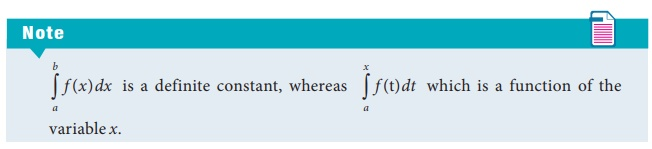Home | | Business Maths 12th Std | The fundamental theorems of Integral Calculus

# The fundamental theorems of Integral Calculus

First fundamental theorem of Integral Calculus, Second fundamental theorem of Integral Calculus

The fundamental theorems of Integral Calculus

## Theorem 2.1First fundamental theorem of Integral Calculus:

If f (x) is a continuous function and F ( x) = xa f (t)dt, then F ( x) = f (x).

## Theorem 2.2 Second fundamental theorem of Integral Calculus:

Let f (x) be a continuous function on [a,b], if F ( x) is anti derivative of f (x) , then

ba f (x)dx = F (b) F (a).

Here a and b are known as the lower limit and upper limit of the definite integral.Tags : Definite integrals , 12th Business Maths and Statistics : Chapter 2 : Integral Calculus - I
Study Material, Lecturing Notes, Assignment, Reference, Wiki description explanation, brief detail
12th Business Maths and Statistics : Chapter 2 : Integral Calculus - I : The fundamental theorems of Integral Calculus | Definite integrals